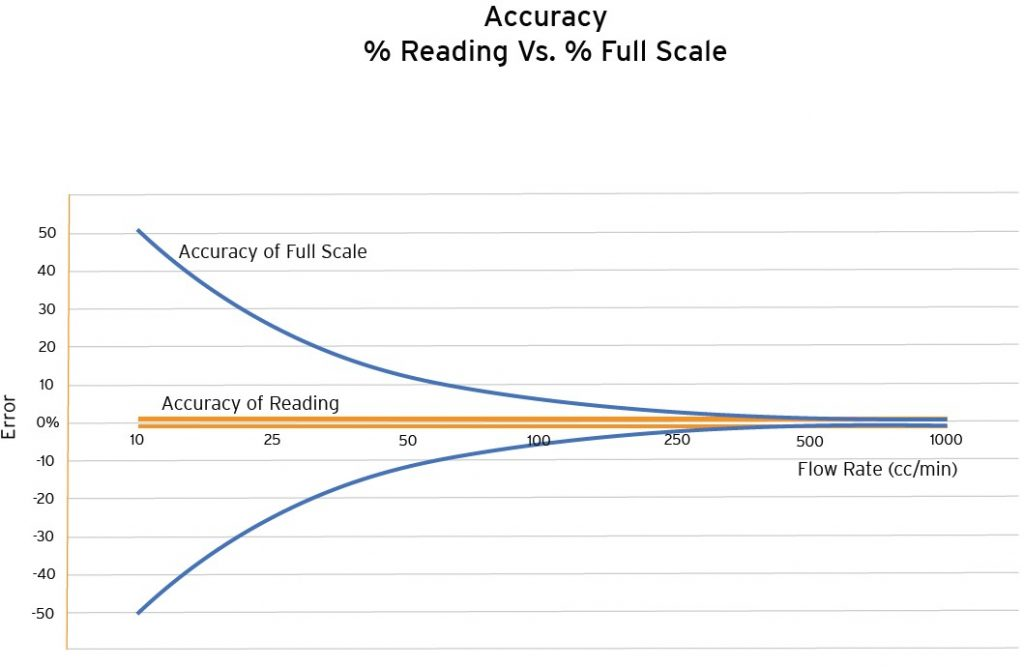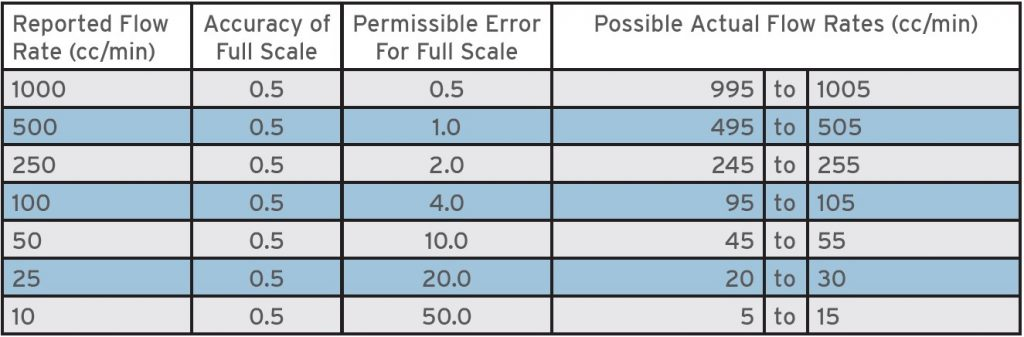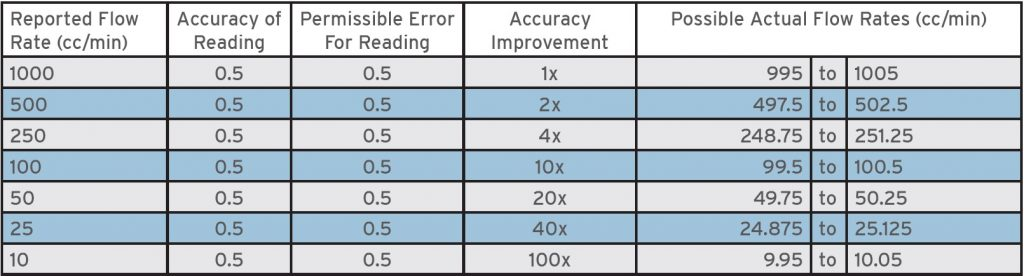# Tech Article – Accuracy of Liquid Flow Meters – % Reading vs % Full Scale

Q: What is the difference between an accuracy claim % of Reading versus an accuracy claim % of Full Scale?

Max: Your true flow rate of the liquid being measured can be a challenging value to determine no matter what type of liquid flow meter you are using. One of the key values to determine how “true” your meter is reporting is to know if the meter’s accuracy performance is based on “% Reading” or “% Full Scale” (also known as Range).

Let’s use a sample flow meter that has a maximum flow rate of 1000 cc/min with a turndown of 100:1, so it can measure a minimum flow rate of 10 cc/min. To focus on the accuracy of the data, we will assume the viscosity, pressure, temperature, flow profile, and all other factors that affect different types of liquid flow meters are fixed and not significant to the results for this explanation.

If this 1000 cc/min meter has an accuracy of 0.5% of Full Scale, the permissible error at each output flow rate changes linearly within the specified turndown range. Here are some sample readings:If this 1000 cc/min meter has an accuracy of 0.5% of Reading, it has a permissible error of ±0.5% across all measurement data being output. Here are the same sample readings:Comparing the readings of the two meters shows that the meter with a stated accuracy of reading provides flow rate data closer to the true flow rate in the system than a meter with a stated accuracy of full scale because the error of the meter is measured based on the current flow rate output rather than the maximum flow rate output.